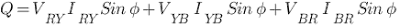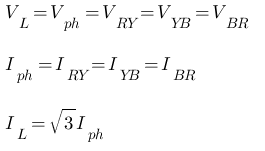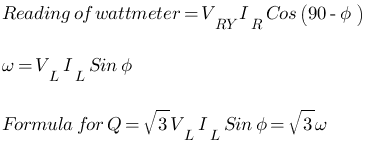# How to Measure 3-Phase Reactive Power of a Induction Motor Using Single Wattmeter

Here we aim to measure the three phase reactive power ‘Q’ absorbed by the given 3Φ induction motor by using single wattmeter. The formula for the reactive power ‘Q’ absorbed by “Balanced Load” is given bywhere,

• Q = Total reactive power absorbed
• VL = Line voltage of supply
• Vph = Voltage per phase of supply
• IL = Line current of supply
• Iph = Phase current of supply
• Φ = power factor angle

The break load on the induction motor can be taken as a balanced load on the I.M. The measurement can be done using the conventional ‘Dynamometer type of wattmeter’. Here the current coil of the wattmeter is connected in the R-phase, the pressure coil is connected between Y-phase and B-phases.

Assuming that, Q = total reactive power.for delta connection,The phase angle between IR and VYB is (90 – Φ)